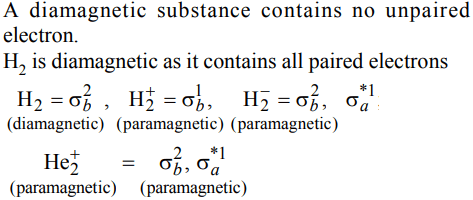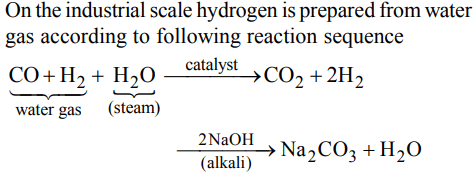## Hydrogen Questions and Answers Part-3

1. The correct order of the O–O bond length in $O_{2},H_{2}O_{2} and O_{3}$   is
a) $O_{2}> O_{3}>H_{2}O_{2}$
b) $O_{3}>H_{2}O_{2}>O_{2}$
c) $H_{2}O_{2}>O_{3}>O_{2}$
d) $O_{2}> H_{2}O_{2}>O_{3}$

Explanation:2. The hydride ion H is stronger base than its hydroxide ion OH. Which of the following reactions will occur if sodium hydride (NaH) is dissolved in water ?
a) $H^{-}\left(aq\right)+H_{2}O\rightarrow H_{3}O^{-}$
b) $H^{-}\left(aq\right)+H_{2}O\left(l\right)\rightarrow OH^{-}+H_{2}$
c) $H^{-}+H_{2}O \rightarrow$   No Reaction
d) None of these

Explanation: H+ (aq) + H2O(l) $\rightarrow$   OH- + H2. Since H- is a strong base it will abstract H+ to form H2O.

3. The component present in greater proportion in Coal gas is
a) $CH_{4}$
b) $CO_{2}$
c) CO
d) $H_{2}$

Explanation: Composition of Coal gas is 45%, H2 , 35%, CH4 , 8%, CO, 4% gaseous hydrocarbons and 8% N2 , O2 CO2 .

4. The O – O – H bond angle in $H_{2}O_{2}$ is
a) 106°
b) 109°28'
c) 120°
d) 94.8°

Explanation: O – O – H bond angle in H2O2 is 94.8°

5. When $H_{2}O_{2}$ is oxidised the product is
a) $OH^{-}$
b) $O_{2}$
c) $O^{2-}$
d) $HO_2^-$

Explanation:6. Some statements about heavy water are given below:
(A) Heavy water is used as a moderator in nuclear reactors.
(B) Heavy water is more associated than ordinary water.
(C) Heavy water is more effective solvent than ordinary water.
Which of the above statements are correct?
a) (A) and (C)
b) (A) and (B)
c) (A), (B) and (C)
d) (B) and (C)

Explanation: (A) and (B)

7. Which one of the following processes will produce hard water ?
a) Saturation of water with $MgCO_{3}$
b) Saturation of water with $CaSO_{4}$
c) Addition of $Na_{2}SO_{4}$  to water
d) Saturation of water with $CaCO_{3}$

Explanation: Permanent hardness of water is due to chlorides and sulphates of calcium and magnesium i.e CaCl2 , CaSO4 , MgCl2 and MgSO4

8. Which of the following species is diamagnetic in nature?
a) $H_2^-$
b) $H_2^+$
c) $H_{2}$
d) $He_2^+$

Explanation:9. Which of the following statements in relation to the hydrogen atom is correct ?
a) 3s, 3p and 3d orbitals all have the same energy
b) 3s and 3p orbitals are of lower energy than 3d orbital
c) 3p orbital is lower in energy than 3d orbital
d) 3s orbital is lower in energy than 3p orbital10. In context with the industrial preparation of hydrogen from water gas $\left(CO+H_{2}\right)$  , which of the following is the correct statement?
a) $CO and H_{2}$  , are fractionally separated using differences in their densities
b) CO is removed by absorption in aqueous $Cu_{2}CI_{2}$  solution
c) $H_{2}$ is removed through occlusion with Pd
d) CO is oxidised to $CO_{2}$ with steam in the presence of a catalyst followed by absorption of of $CO_{2}$ in alkali